### Request a Demo

Which industry are you working in?
Consumer Goods and Retail
Telecom and Internet
Consumer Software
Other industry
What do you want to work on?
Pricing
Portfolio
Data analytics
Annual commercial planning
Product
Other
How much revenue did your company generate last year?
< \$50M
\$50 M > \$1B
>\$1B

## Success

Thank you for registering for our upcoming event. You will receive a Zoom invite by email soon.

## Application sent

Thank you for sending your application.

# The Truth about Price Elasticity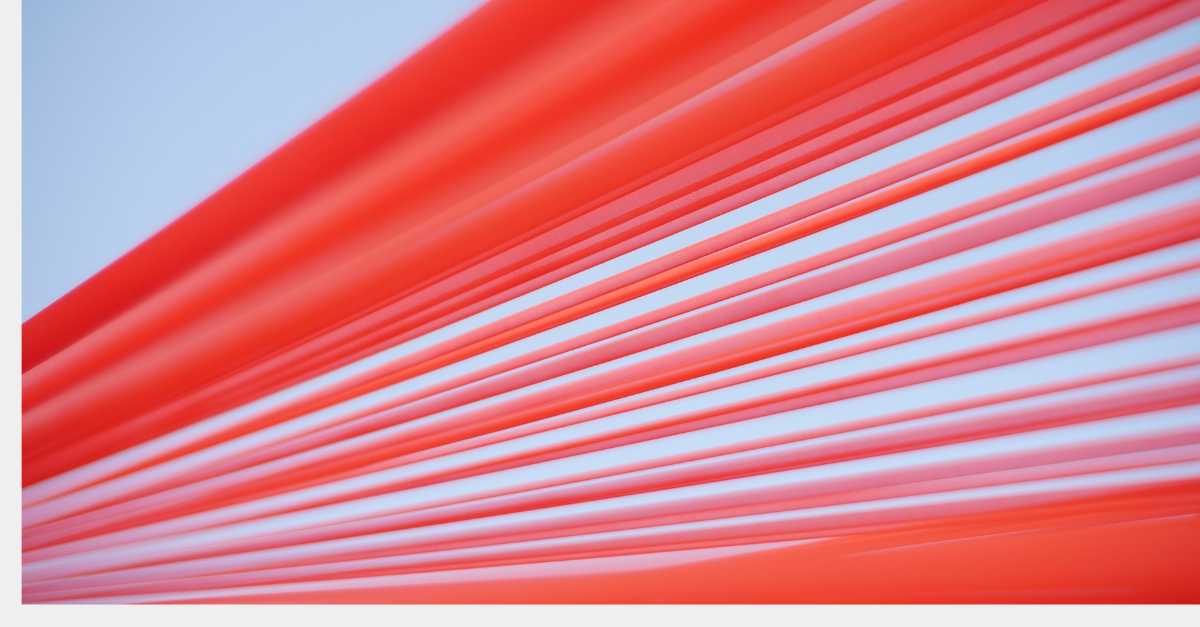# ''Price elasticities are like sausages; everyone likes them, but it’s better not to see them being made.''

The most important thing in pricing is to understand the customer. Measuring price elasticity is a widely used method to do so. More specifically, price elasticity is measured to answer the question: “How have customers adapted their purchasing behavior to price changes in the past?” From this it shall be deduced how to set prices in the future in order to achieve pricing goals (e.g. optimize profit, revenue or market share).

In our last elasticity blog we discussed the general concept of price elasticity and saw how to set prices to maximize profit or revenue given a precisely measure price elasticity. In this blog, we will focus on the measurement of price elasticity itself and the reliability of results.

#### Recap

We operate the only lemonade stand in town and offer exactly one product: lemonade.

We tested prices from \$0.00, where we “sold” 1,000 units per month, to \$2.00, where no one bought from us any more (Graph A). The corresponding elasticity is shown in Graph B.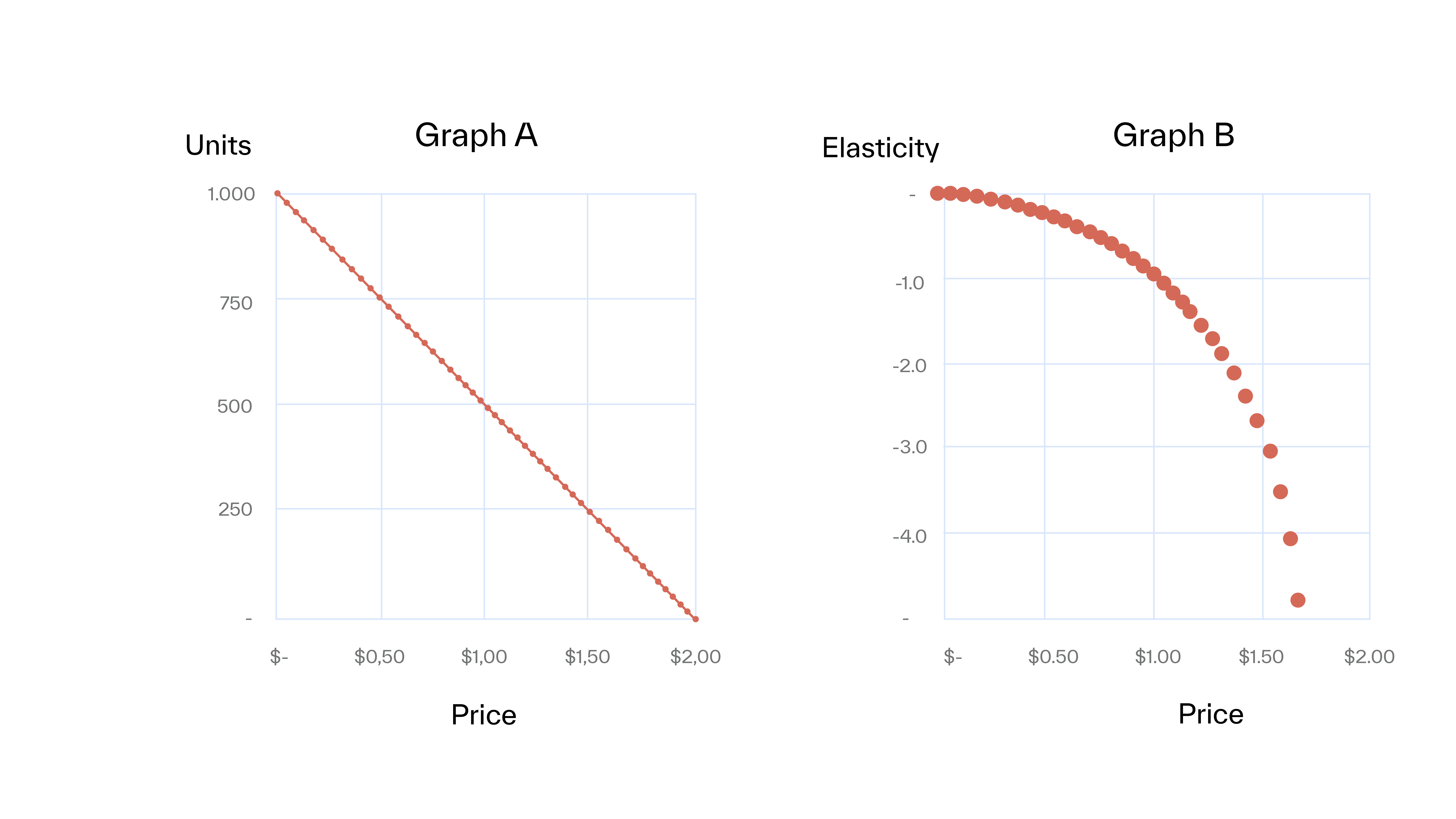Note, that the elasticity is not constant but changes with price. Although this is true in reality, we need to assume a constant elasticity over a limited price range to be able to determine it by linear regression*.

We made two more assumptions in the initial scenario that are oversimplified and need to be changed:

1. We tested the full price range (from \$0.00 to \$2.00) in steps of \$0.05. Unless one works for a very relaxed CEO / CFO, this will not be possible. If you have an experimental mindset, you will test prices in a much narrower range (say +/- 10%). Often, prices are not intentionally “tested,” but come from more or less thought-out rules.
2. Most importantly, there is zero noise in the data. In other words: price is the only factor that influences the purchasing decision. Not weather, not vacations, not concerts, football games or pure chance. Obviously, that’s not realistic.

Let’s assume that we have operated our lemonade stand for one year and we haven’t really cared much about pricing. We simply did “Cost-Plus” and calculated our price by doubling the cost to get a margin of 50%. We adapted our prices approximately every other week to changing costs. To determine the price elasticity we plot our 48 price/units combination and fit the demand function on the data to determine price elasticity**. The result is shown in Graph C.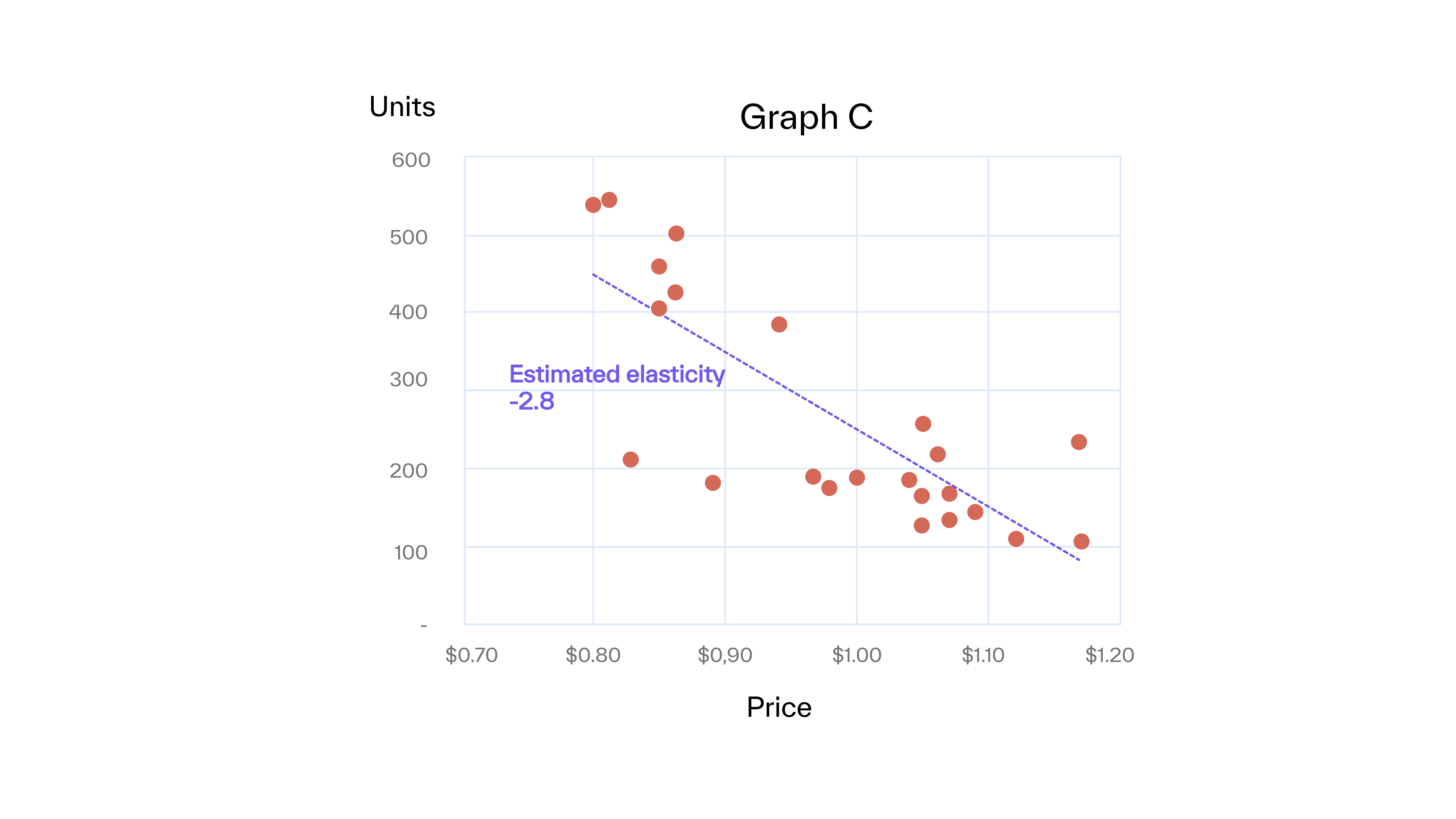The units no longer lie on a straight line as in Graph A’s ideal world. Obviously, there are other influences on buying behavior than price. These other factors show up in Graph C as “noise”. This noise can be attributed to two main sources: systematic and random noise.

#### Systematic noise

Systematic noise can be explained –in principle– by external circumstances. For our lemonade stand we thought hard and came up with the explanations shown in Graph D. In theory, all influencing factors could be considered as extensions to the price-demand-function. In reality, this may work for obvious and reliable factors, such as season or vacations, while many sources will be unknown (like a private party) and/or the corresponding data is not available or trustworthy. And even if all data would be available, we still need to translate an influencing factor (e.g. temperature) into the proper mathematical term. Also, the interconnection of different influencing factors needs to be considered.

For example, how would you consider an August (higher consumption), with the first 2 weeks vacation time (lower consumption), with higher than normal temperature (higher consumption), but at lot of rain (lower consumption) that has mainly fallen at night (higher consumption)? Therefore, if in doubt, it is better to leave sources out. Otherwise the model will be over-fitted leading to substantially worse results than leaving potential sources out in the first place. As John von Neumann once said: “With four parameters I can fit an elephant, and with five I can make him wiggle his trunk.”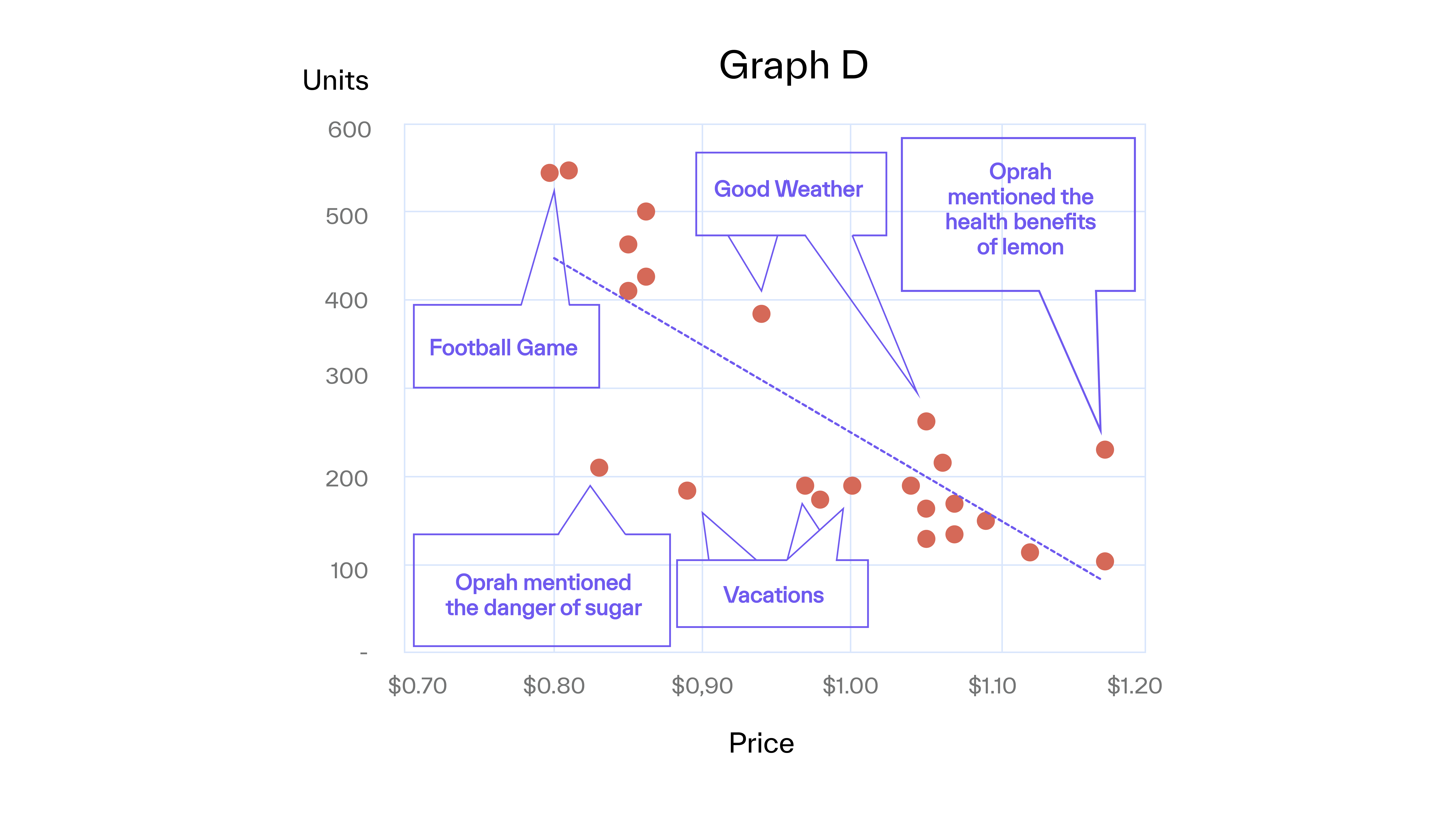### Random noise

Random noise arises from stochastic fluctuations and cannot be predicted. The number of potential buyers who feel like having lemonade today (before they even consider prices) is a source of random noise. There is no way to eliminate or correct for random noise, except by increasing the sample size.

Now, since we have considered all the noise, we want to know how reliable this result is before we change our prices. In other words: how much noise is acceptable for valuable price decisions? Unfortunately, the answer is: not much.

Table E shows the level of noise and the corresponding reliability of elasticity and derived prices***.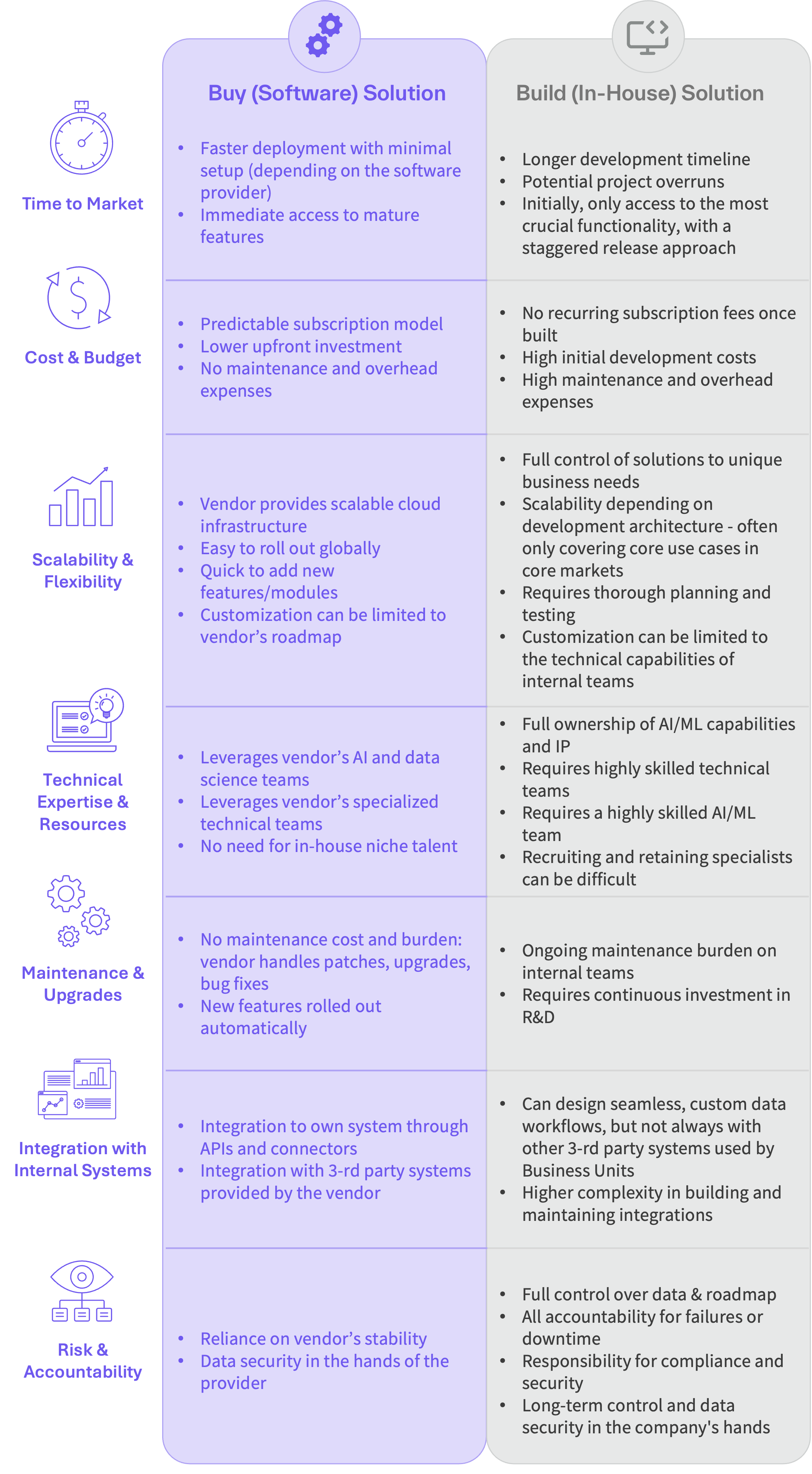Let’s look at the “10% Noise” example to understand better what happens here. The 10% noise means that 10% of variation in demand cannot be explained by price. This is actually very little. If we sell 100 units on average per month, this corresponds to an unexplainable deviation of 10 units. This could be caused by a BBQ in the neighbourhood (+10) or a regular customer’s vacation (-10).

For everyone who has ever determined elasticities with real data, the 10% and 25% noise graphs look very smooth. Even the 50% noise graph looks much smoother than most data seen in real life. But even 10% noise lead to considerable uncertainty in elasticity. From 10,000 simulations, 95% of determined elasticities are within -2.5 and -1.5 (where the true elasticity is -2). If we determine the optimum price based on these elasticities, our average error is 11%. For 25% noise, the error is 36%. In other words, if the ideal price is \$1.00, the price determined from elasticity measurement is on average as bad as \$1.36 (or \$0.64). This is hardly acceptable.

Understanding the customer is at the core of good pricing. Leveraging sales data is often the only way to do this. As we saw, elasticity is a poor approach for it.

* The only price demand function – our hypothesis of the true relationship between price and demand – with constant elasticity is

Q = α ∗ P^β (Q: demand; α: constant; P: price; β: elasticity).

It has constant elasticity (e.g. the elasticity does not depend on price) since

Εlastictiy = ∂Q/Q / ∂P/P = ∂Q/∂P ∗ P/Q = β ∗ α ∗ P^(β-1) ∗ P/Q = β ∗ Q/Q = β.

To determine price elasticity the function is logarithmized

log(Q) = log(α) + log(P) * β.

If we plot log(Q) over log(P), we get the elasticity β as the slope.

** Actually we do a linear fit on the logarithms of price and demand (see *).

*** To generate the test dataset, 24 periods have been simulated. Each period has one price and corresponding demand. The price for every period was randomly drawn between \$0.90 and \$1.10. The corresponding “Ideal World” demand has been calculated according to the price demand function Q = α ∗ P^β (with alpha = 500, and true elasticity β = -2). To simulate noise, two random numbers have been added to these “Ideal world” sales.

1. Random noise, drawn from a binomial distribution, which cannot be controlled for.

2. Systematic noise of X% by multiplication of the “Ideal World” sales with a random number between 1-X and 1+X (e.g. 10% noise by multiplication of a random number between 0.9 and 1.1). The logarithmized price demand function has then been fitted on this dataset to estimate the elasticity β.